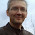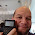## Thursday, June 23, 2016

### Programming with the Radio Shack EC-4004

Programming with the Radio Shack EC-4004

(Another milestone: this is blog #600! I can't thank you all enough!)

To see my retro review of this calculator I did two years ago, click here:

The Radio Shack EC-4004 calculator’s programming mode is AOS (algebraic operating system).  Rarely you see a calculator with AOS any more (think the Casio fx-260 and Texas Instruments TI-30A), but it used to be prominent operating system with RPN.   In AOS, the uniary functions (trigonometric, logarithmic, factorial, for example) are entered after you enter the number.  Hence:  14 [ln] calculates ln(14) ≈ 2.639057329.

The storage of the EC-4004 is a mere 38 steps which can be distributed into two program slots.  That is not a lot.  Furthermore, if you enter numeric constants, each digit counts a step.  Remember, the display does NOT display the key, so be careful when entering programs.  There are a few program commands:

RTN ([INV] [ 9 ]):  Returns program control the first step (beginning).

X ≤ M ([INV] [ 8 ]):  If the display is less than or equal to M, return to the first step.  Otherwise, go to the next step.

X > 0 ([INV] [ 7 ]): If the display is greater than 0, return to the first step.  Otherwise, go to the next step.

ENT ([RUN] in learn mode):  Prompt for a number.  The next step is to put a “placeholder” number as long as it will be valid in calculations.  Since each digit counts as a step, try to use placeholder numbers like 0, 1, or 2.  I’m not sure if the placeholder number is merged with ENT.  You can instead, use Kin or Min to store the entry in memory.

HLT ([INV] [RUN]): Halts the program to display immediate results.  Think of it as the PAUSE command.

X<>K ([INV] [Kout]): Exchange with a memory register.  The EC-4004 has six memory registers, plus one additional independent memory register (M).

[Kin] and [Kout] is your store and recall, respectively.

On to the list of sample programs!  Anything after double slashes (\\) is a comment. The hashtag (#) stands for placeholder number.

Area of a Regular Polygon

Formula:  (n*s^2)/(4*tan(180°/n)), n = number of sides, s = side length

MODE,  4 \\ sets Degree mode
ENT \\ prompt for n
#  \\ enter a placeholder number
Min \\ store in M
*
ENT \\ prompt for s
#  \\ placeholder number
X^2
÷
(
4
*
(
180
÷
MR \\ recall n
)
TAN
)
=

Test 1:  n = 6, s = 2.6,  result 17.56299519
Test 2:  n = 10, s = 3.87, result 115.2353964

Sum of f(x)

In particular, Σ x^2 + 1 from x = 1 to M.  M is the upper limit.  You can adapt this to include any f(x), but remember you’ll only have 26 steps to work with for f(x).  Your variable for f(x) is register 1 (use [Kout], 1)

Before running, store the upper limit minus 1 in M, 0 in both registers 1 and 2.  (0, [ Kin ], 1; [ Kin ], 2).  Register 1 is your counter, register 2 is your sum.   In Summary:

M = upper limit – 1, Register 1 = 0, Register 2 = 0

Kout 1  \\ recall register 1 and add one
+
1
=
Kin 1
X^2 \\ f(x) starts here
+
1
=   \\ end f(x) with equals
+   \\ add result to register 2
Kout 2
=
Kin 2
Kout 1 \\ put register 1 in the display
X≤M   \\ is X≤M? If so, go to the beginning
Kout 2 \\ display sum

Test 1:  If the upper limit is 5, store 4 in M.  Result:  60
Test 2:  If the upper limit is 8, store 7 in M.  Result:  212

Payment of a Loan (with no balloon)

Formula:  PMT = PV / ((1 – (1 + r)^-n)/r)

Store the following amounts before running:
K1 = PV, present value or loan amount
K2 = n, number of payments.  Example: For 30 years for monthly payments, store 360 in K2.
K3 = r, periodic interest rate as a decimal.  Example: For 6% compounded monthly, enter 0.06/12 in K3.

Kout 1 \\ recall PV
÷
(
(
1
-
(
1
+
Kout 3  \\ recall r
)
X^Y
Kout 2 \\ recall n
+/-
)
÷
Kout 3
)
=

Test 1:  K1 = 200,000, K2 = 360, K3 = 0.055/12.  Result:  1,135.58
Test 2:  K1 = 234,000, K2 = 360, K3 = 0.038/12.  Result:  1,090.34

Easy Traverse Calculation

Calculates the new point knowing the original coordinates, direction, and angle of travel.  The angle 0° comes from due east and rotates counterclockwise (see diagram below).

Store before hand:
K1 = original coordinate easting (E, x)
K2 = original coordinate northing (N, y)

Distance is stored in K3 and angle is stored K4.  At the completion of the program, the new coordinates are stored in K1 and K2, respectively, to allow chain calculations.

MODE 4 \\ degrees mode
Kout 1
+
ENT \\ prompt for distance
# \\ placeholder number
Kin 3
*
ENT \\ prompt for angle
# \\ placeholder number
Kin 4
COS
=
Kin 1
HLT \\ display new easting coordinate
Kout 2
+
Kout 3
*
Kout 4
SIN
=
Kin 2 \\ display new northing coordinate

Test:  E0 = 10,000,  N0 = 10,000
Given distance = 110, angle = 126°,  E1 ≈ 9,935.343, N1 ≈ 10,088.992
Continuing,
Given distance = 150, angle = 30°, E2 ≈ 10,065.247, N2 ≈ 10,163.992

Measuring Dew Point (in degrees Celsuis)

W = 237.3 * V/(1 – V)
V = (ln H + (17.27*C)/(237.3+C))/17.27

Where:
H = relative humidity, store as a decimal (Example:  for 55.8% store 0.558 in K1)
C = temperature in degrees Celsius

Store before hand:  H in register 1 (K1), C in register 2 (K2).

This program illustrates a perfect example of the effect of the limited programming space.  During the calculation, 17.27 and 237.3 are stored in K3 and K4, respectively to save program space.  And believe me, we’ll need every bit of it.

Kout 1 \\ H
LN
+
17.27
Kin 3
*
Kout 2 \\ C
÷
(
237.3
Kin 4
+
Kout 2
)
=
÷
Kout 3
Min // store V in M
*
Kout 4
÷
(
1
-
MR
)
=

Remember K1 = H, K2 = C
Test 1:  H = 51% (0.51), C = 27°C, Result ≈ 16.0003°C
Test 2:  H = 46% (0.46), C = 85°F = 29.44444444°C, Result ≈ 16.6110°C

Chebyshev Polynomial of the First Kind, for -1<x<1

Formula for -1<x<1:  T_n(x) = cos(n * acos x)   (source: Wolfram, http://mathworld.wolfram.com/ChebyshevPolynomialoftheFirstKind.html, see line 44)

ENT \\ prompt for x
#  \\ placeholder number
COSˉ¹
*
ENT \\ prompt for n
#  \\ placeholder number
=
COS

Test 1:  T_2(0.25) = -0.875  (x = 0.25, n = 2)
Test 2:  T_3(-0.68) = 0.782272  (x = -0.68, n = 3)

That is a small collection of programs for the EC-4004.  If you want me to do more, let me know.  Until then, have a great day everyone and stay safe!

Eddie

This blog is property of Edward Shore, 2016

#### 5 comments:

1.Hello Eddie!

Thanks for sharing these programs! I think I'll convert this post to pdf and put into my limited program library of these "blind programmable calculators" (as we can found these calcs on Viktor Toth's site).

My favourite is the CASIO 50F with 23 preprogrammed equation and physical constants. I guess some of above impossible to convert to my calculator, because it has really limited capacity: only 29 steps.

If you are interested and do not fear Hungarian language you can find some programs for these calculators here:

Log(x) for any base and a DEG-RAD/RAD-DEG converter: https://groups.google.com/d/msg/szamologep/u2BFOwqeD6E/pIemEiApKh4J

Guess a number game: https://groups.google.com/d/msg/szamologep/u2BFOwqeD6E/Dx5b8uh8NrMJ

f(x)=0 solver and an application: inverse Normal distribution with the built-in Normal distribution equation (pdf! page 10~14): https://drive.google.com/open?id=0B1AqWV7mL061WkYyRnJCTTNVblU

Csaba

1.Thank you! 29 steps isn't much. Neither is 38 to be honest. I just purchased a TI-55 III (it's on its way) and the room is only 54 steps (without memory registers?).

Eddie

2.Hello Eddie!

Just check the page 22 of this manual:
http://www.usersmanualguide.com/manuals/casio/FX-10F_FX-50F.pdf

You can find more information about the "placeholder number".

Csaba
-----
http://t-500.blogspot.hu/

1.Thank you! I downloaded the instruction book and will check it out.

3.Hi boys n girls,
up to now I thought that my Casio FX-502 P had not so much memory with it's 256 bytes. But now I see it was a memory gigant compared to the EC-4004 (yes, they were looking very familiar). And my FX-602P has monstrous 512 bytes of ram. No idea ever how to fill it. :-)

### Update: HP Prime Connectivity Kit and Emulator

Update: HP Prime Connectivity Kit and Emulator The HP Prime Connectivity Kit and Emulator have been updated.  The version number is 14592 ...